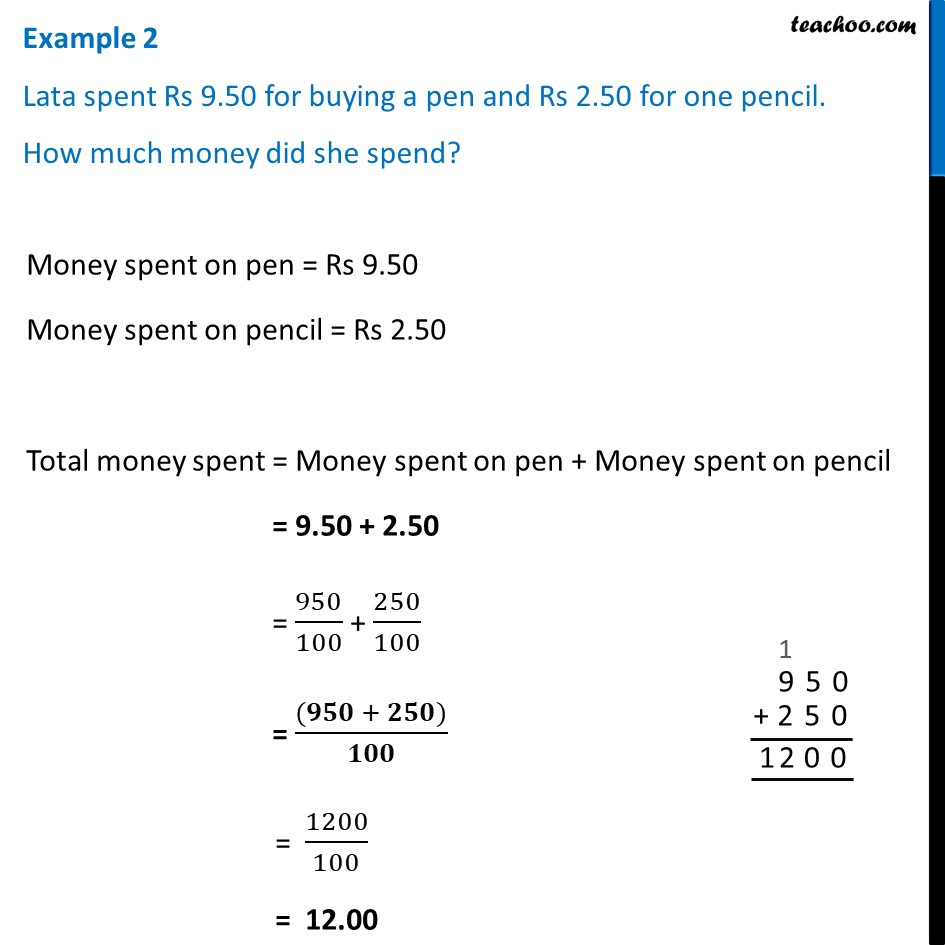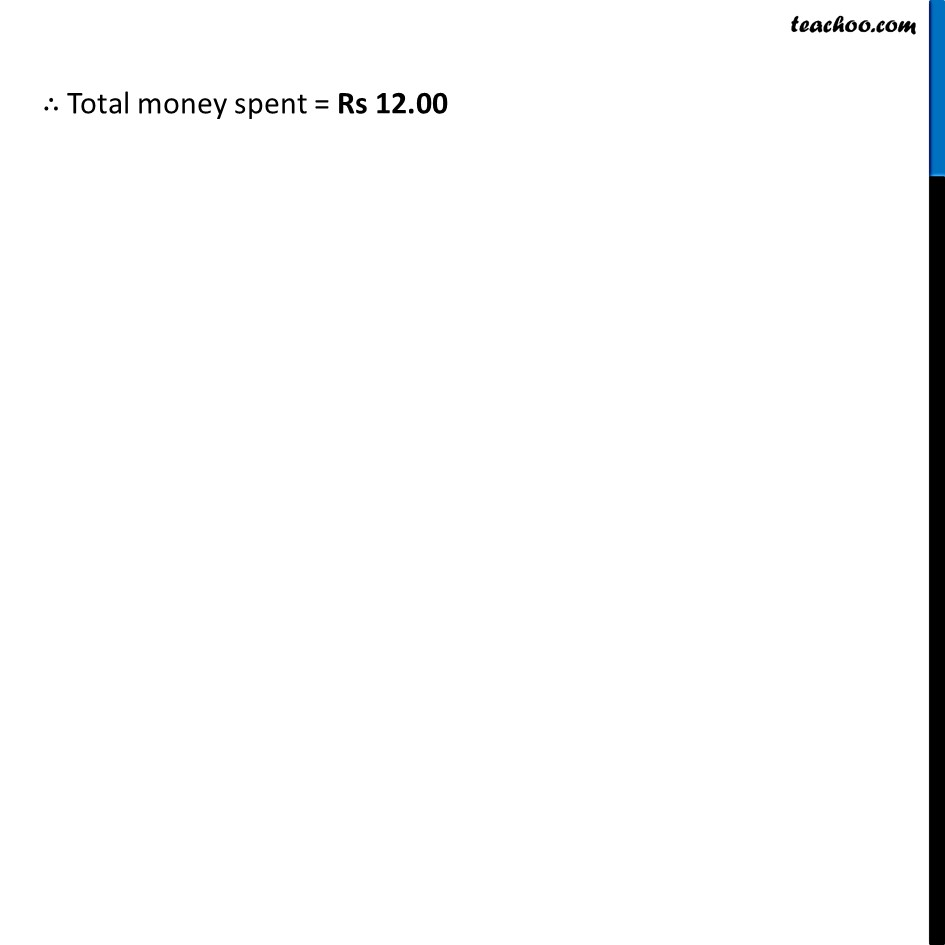Examples

Chapter 8 Class 6 Decimals
Serial order wiseLearn in your speed, with individual attention - Teachoo Maths 1-on-1 Class

### Transcript

Example 2 Lata spent Rs 9.50 for buying a pen and Rs 2.50 for one pencil. How much money did she spend?Money spent on pen = Rs 9.50 Money spent on pencil = Rs 2.50 Total money spent = Money spent on pen + Money spent on pencil = 9.50 + 2.50 = 950/100 + 250/100 = ((𝟗𝟓𝟎 + 𝟐𝟓𝟎))/𝟏𝟎𝟎 = 1200/100 = 12.00 ∴ Total money spent = Rs 12.00